# Scalene Triangle - Definition with Examples

The Complete K-5 Math Learning Program Built for Your Child

• 40 Million Kids

Loved by kids and parent worldwide

• 50,000 Schools

Trusted by teachers across schools

• Comprehensive Curriculum

Aligned to Common Core

What is a Scalene Triangle?

A triangle is a polygon made up of 3 sides and 3 angles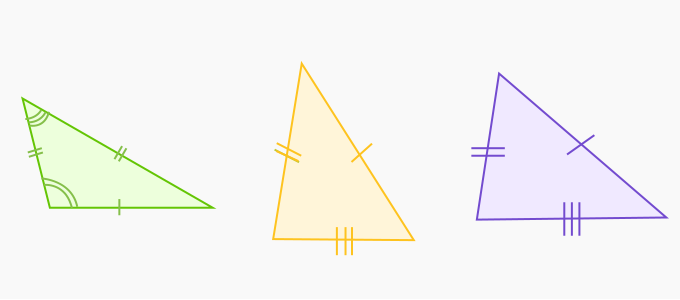We can classify triangles according to the length of their sides.

(i) Equilateral triangle: If all sides of a triangle are equal, then it is called an equilateral triangle.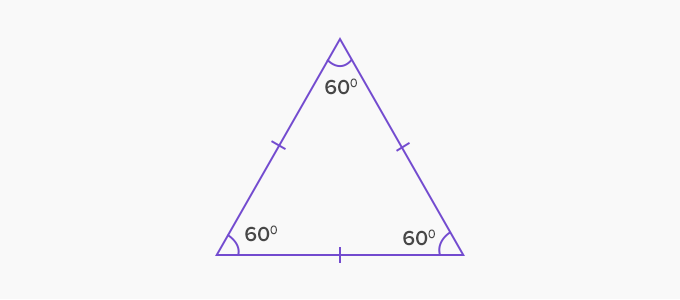(ii) Isosceles triangle: If two sides of a triangle are equal, then it is called an isosceles triangle.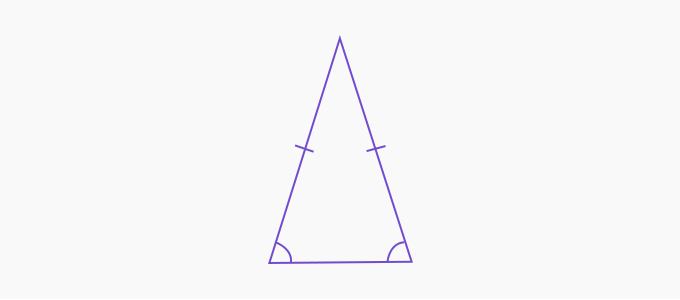(iii) Scalene triangle: if none of the three sides of a triangle are equal to each other, it is called a scalene triangle.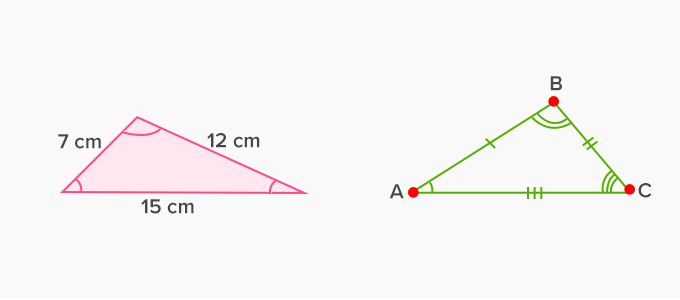Properties of the scalene triangle:

 All angles of a scalene triangle are unequal. A scalene triangle has no line of symmetry. The angle opposite to the longest side would be the greatest angle and vice versa. All sides of the given scalene triangle are unequal.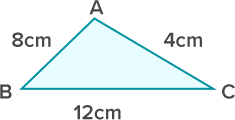The given triangle cannot be divided into two identical halves. There is no line of symmetry.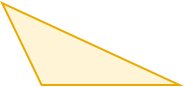In this triangle, angle E will be the greatest angle as it is opposite to the greatest side GO and angle O will be the smallest angle as it is opposite to the smallest side GE.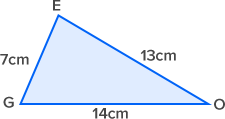A scalene triangle can be acute-angled or obtuse-angled or right-angled.

 Obtuse scalene triangle Acute scalene triangle Right scalene triangle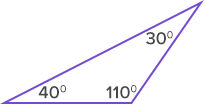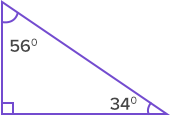Real life examples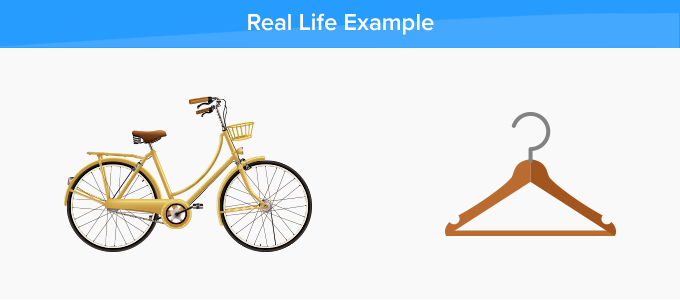Fun Facts The term scalene triangle is derived from Late Latin ‘scalēnus’ and from the Ancient Greek word ‘σκᾰληνός’ which means unequal or uneven.

Won Numerous Awards & Honors